# Theoretical study of rabi oscillations in Dirac-weyl fermionic system

N/A
N/A
Protected

Share "Theoretical study of rabi oscillations in Dirac-weyl fermionic system"

Copied!
197
0
0

Full text

4 1.3 The first figure shows the Wigner-Seitz primitive cell obtained from the direct lattice of graphene. 137 5.3 The plot shows the anomalous Rabi frequency versus energiz. 5.50)), it depicts the existence of anomalous Rabi oscillation at a zero-photon limit, the parameters are plotted in the units of ω and λ = 1.

## Graphene

• Geometry of Graphene
• Honeycomb Lattice (Graphene) i.e. Nearest-Neighbor (NN)
• Pseudospin in Graphene
• Graphene Electronic and Transport Properties
• Graphene Optical Properties

Due to hybridization, the carbon atom has an sp2 hybridized orbital (orbitals formed from one and two orbitals). σ bonds are formed when the 2s, 2px, and 2py orbitals mix together. The Hamiltonian of the closest tight bond of graphene can be written as (we use such units that . ~= 1).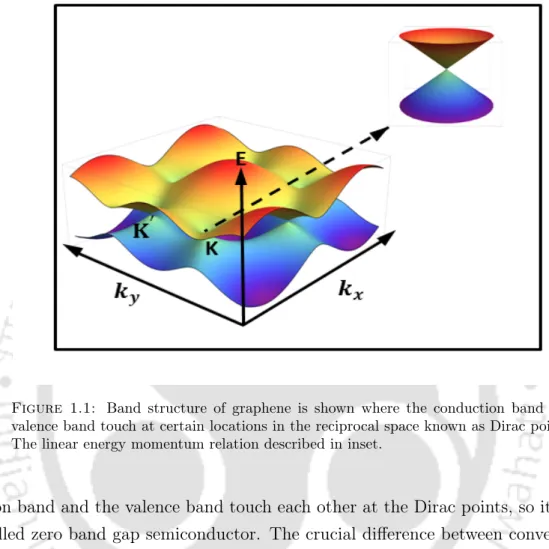Figure 1.1: Band structure of graphene is shown where the conduction band and valence band touch at certain locations in the reciprocal space known as Dirac points.

## Topological Insulator

### Honeycomb Lattice (Graphene) i.e. Next Nearest Neighbors

• Potential Energy (Breaking Inversion Symmetry) . 24

In the absence of the complex NNN jumps, the gap is 2M at K and K0, and the system becomes a trivial insulator at half-fill. There is a band crossing in the system (either at K or K0 depending on the sign of M and t0sinφ ) at the transition point and the system is not an insulator.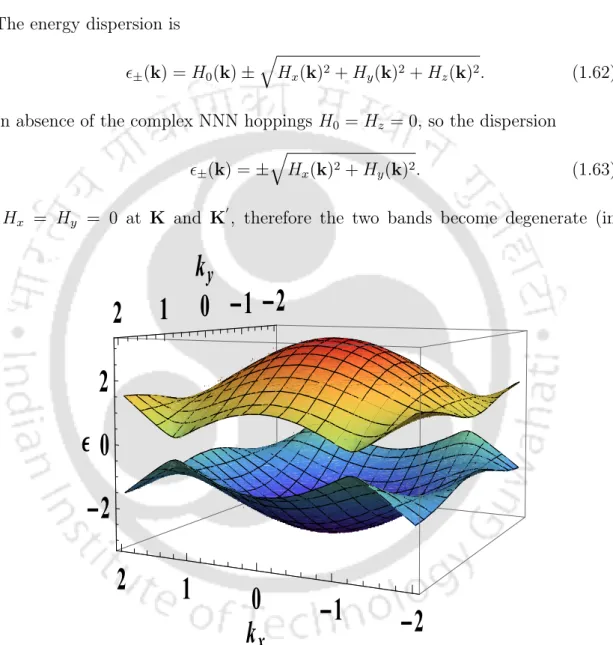Figure 1.5: The plot between energy dispersion ± and k x and k y for both bands in the first Brillouin zone.

### Kane-Mele Model

When the time-reversal symmetry is broken, edge states disappear and the system returns to a trivial insulator. This means that there are two types of insulators: no edge states (trivial) or 1 pair of edge states (topological).

### Terahertz and Infrared Study of Topological Insulators

Because of the residual conductivity associated with the bottom of the bulk conduction band, reflectance measurements on TI single crystals present the same problems as transport measurements for signal detection of surface states. A major advantage of THz spectroscopy is that it can detect topological surface states and distinguish surface states from bulk states in topological insulators.

## Weyl Semimetal

• Dirac Fermions
• Weyl Fermions
• Possible Realization of Weyl semimetals
• Chiral Anomaly in Weyl semimetal
• Electronic Properties of Weyl Semimetal
• Optical Properties of Weyl Semimetal

The simplest realization of the three-dimensional Weyl semimetallic phase is proposed in topologically normal insulator heterostructures. Spin splitting of surface states is achieved due to doped magnetic impurities in the layers of the topological insulator.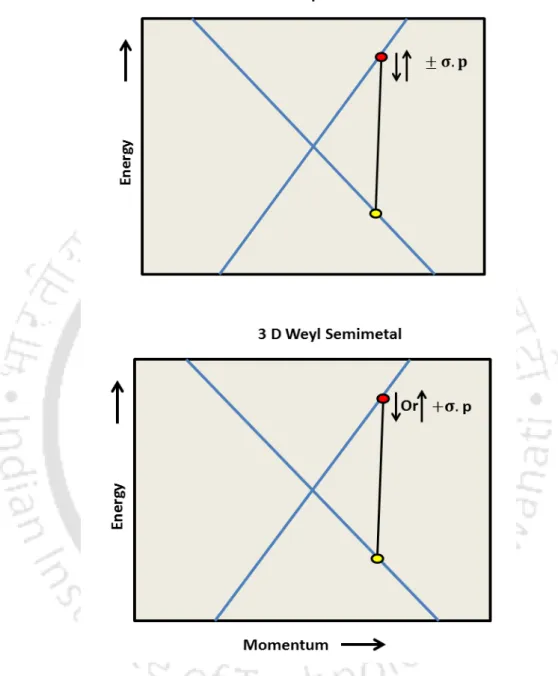Figure 1.6: Band structure comparison between 2D graphene and 3D Weyl Semi- Semi-Metal system

## Applications

### Graphene

When we use graphene on the surface of the anode in lithium-ion batteries, lithium ions start to adhere to the anode substrate due to defects in the graphene sheet (introduced through heat treatment). The ease with which large areas of graphene sheets can be produced means they can be used in the manufacture of capacitors.

### Topological Insulator

Due to shielded surface/edge states in TI, there is absence of decoherence in the spin current. The advantage of the unique surface electrons of topological insulators can be used in the future electronic device for energy utilization.

### Weyl Semimetal

From topological field theory, the coefficient of the axion term (eq.(1.90)) indicates the value of the Hall conductance . By measuring the surface Hall conductance in the strong topological insulator, this confirms the topological robustness and also the axion electrodynamics.

## Rabi Oscillations in Two-Levels

We consider zero detuning: ω=ω0where one sees the well-known Rabi oscillations between the ground and the excited state of the two-level driven system. The frequency ΩRabi depends only on the intensity of the applied optical field and is independent of the applied frequency.

## Introduction

With the advent of new Dirac-Weyl fermionic systems in condensed matter, many works have appeared comparing and contrasting conventional semiconductors/metals and Dirac-Weyl fermionic systems. We want to see if anything new can be learned by studying Rabi oscillations in these Dirac-Weyl systems.

## Problem Formulation

In this chapter, we reported a new approach that is essentially an advance of RWA, which we called the "asymptotic rotating wave approximation (ARWA)". We have studied this phenomenon as well as normal Rabi oscillations close to resonance and verified the claims numerically.

## Weyl Semimetal Hamiltonian in Second Quantized Form

We will obviously obtain the same results using the other extreme choice, namely - the length meter, i.e. using the tight binding approximation, the low energy Hamiltonian of Weyl metals in momentum space with hopping and interaction with electromagnetic radiation (in the radiation meter with vector potential A(t)) in second quantized form.

## Rabi Oscillations in Weyl

### Off-Resonance Case (ARWA)

Here, ΩARW A(p) is the oscillation frequency of the amplitudes of observables, such as polarization, densities, current, etc. The frequency of the observables, such as current, is twice the frequency of the wave function.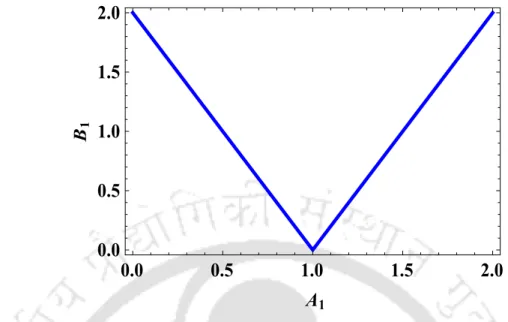Figure 2.1: The plot belongs from eq.(2.22), depicts the anomalous Rabi frequency versus the component of the wave vector p z upon setting p x = 0 and p y = 0 where A 1 = v F p z ω

### Resonance Case(RWA)

In Weyl metals, the anomalous Rabi frequency is peculiar and shows a significant deviation from the form of this quantity seen in the graph. More interestingly, setting px =py = 0 causes the conventional Rabi frequency of the Weyl metal.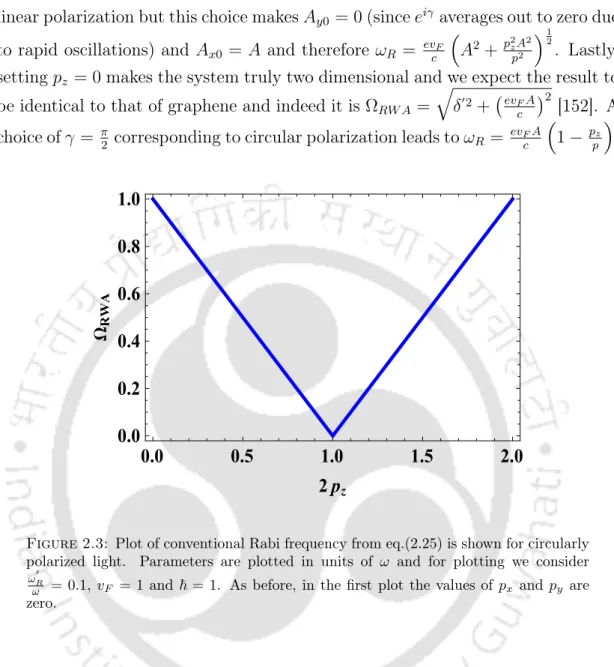Figure 2.3: Plot of conventional Rabi frequency from eq.(2.25) is shown for circularly polarized light

## Fully Numerical Solution

Indicating that the Rabi frequency at conventional resonance is zero and close to it, the dispersion relation of the quanta of conventional Rabi oscillations is linear rather than parabolic, indicating the presence of massless modes. The fast oscillations have a frequency equal to the frequency of external radiation, the time is in the unit 2πωω2.

## Surface States in a 3D Topological Insulator

The 3D plot at the bottom (equation (2.32)) shows the massless Dirac-like cone of the anomalous Rabi frequency at a shifted point in the wave vector space defined by the Zeeman energy scale. This equation (2.33) shows that the modes are enormous even in the absence of the Zeeman term.

## Conclusions

The qualitative effect includes making the anomalous Rabi modes massless if the Zeeman term is absent. When everything else remains fixed (including pump-probe delay), this plot exhibits oscillations with a frequency equal to the anomalous Rabi frequency.

## Appendix

### Methods for Obtaining Anomalous Rabi Frequency

• Current Density Method
• Wave Function Method
• Fourier Transform method
• Numerical Simulation Method

The energy–momentum relationship for graphene shows a linear spread near the six corners of the two-dimensional hexagonal Brillouin zone. Single-layer graphene on a substrate lacks inversion symmetry in a direction perpendicular to the plane of the graphene sheet.

## Problem Formulation

By measuring the Rabi frequency and the Bloch-Siegert shift for both the one- and three-photon cases, it is shown that these coherent phenomena can be observed in a purely classical system. Based on this literature, it is fair to say that the Bloch-Siegert shift is an interesting problem to study.

## Bloch-Siegert Shift in a Two-level System

So the Rabi frequency which in the resonant case was small compared to ω crosses over to a frequency which is of the same order as the external frequency. We study the nature of the Bloch-Siegert shift by first assuming a strictly linear dispersion followed by a more realistic and logically consistent calculation that includes non-linearities in the dispersion.

## Bloch-Siegert Shift in Graphene - Linear Dispersion

This expression is identical to what can be found in the textbooks (see e.g. Allen and Eberly. Therefore, in the ARWA case for isotropic graphene we do not have a Bloch-Siegert shift, but simply a change in the effective Rabi -frequency.

## Bloch-Siegert Shift in Graphene - Band Curvature Effects

We need to shift by ω to extract the Rabi frequency associated with the single harmonic in the external field (the sequence below required six iterations of eqn.(3.29) to obtain). This is the simplest case and leads to the following results for the conventional and anomalous Rabi frequencies (δ2 =|zk|ω). ii) No non-linearities in the dispersion (q = 0) but frequency doubling effects included (ω <∞, see earlier section). iii) Leading non-linearities in the dispersion included (q 6= 0), but frequency doubling effects ignored (ω.

## Bloch-Siegert Shift in Graphene - Rashba Interaction

At low energy there is a change in the topology of the bands near the Dirac points due to trigonal twisting. We note that the origin of the anisotropy is in the expression for the eigenvalue of the energy Ek.

## Results and Discussion

The ARWA Rabi frequency with nonlinearity and frequency doubling included effectively becomes zero at a shifted Bloch-Siegert value of the wavenumber. The second figure is a plot of Rabi anomalous frequency, including frequency doubling and nnn jumping, but no Rashba effect.

Conclusions

## Appendix

Derviation of Bloch Equation in Graphene

### Derviation of Band Curvature Effects in Graphene

3acc ˆj and the B lattice also contain carbon atoms at sites. 3.63) From the above equation, the nearest neighbor selection will be. We can write the above equation in the form g(k) = eipy. 3.74) The Hamiltonian is (the phase factor eiπ6 can be absorbed into the definitions of a and b).

### Conventional Way (Old Approach) of Bloch-Siegert Shift in

In this chapter we have described pump probe spectroscopy used to justify the phenomenon of anomalous Rabi oscillations  - a central idea in this thesis. Using the pump-probe experiment, incoherent optical properties such as optical dephasing and relaxation of band electrons in semiconductors have been studied [48, 49].

## Problem Formulation

### Pump-Probe Spectra in Graphene

The short relaxation τ1 is due to scattering within the carrier-carrier band, which produces quasi-equilibrium states with a Fermi-Dirac distribution. Then there is a decrease in the transmission coefficient; it comes into the plot due to scattering within the carrier-carrier band and within the carrier-phonon band.

Pump-Probe Spectra in Topological Insulator

### Pump-Probe Spectra in Weyl Semimetals

This causes dissipation in the quantum system, which manifests itself as a non-uniform time evolution of the wave function of the quantum system. This is usually done by Lindblad's super-operator method , which modifies the evolution equation of the density matrix of the quantum system.

## Dephasing in Open Quantum Systems: The Lindblad Superoperator 103

### Pump Equation

• Case I: For Time t < 0
• Case II: For Time 0 < t < t pu
• Case III: For Time t > t pu

During this time, the pump field is switched on and the wave function evolves as follows. Furthermore, it is the case that Λ(t → ∞) = 1, which ensures that long after the pump pulse is turned off, the wave function returns to the equilibrium form. 4.9) Now we verify probability conservation.

• Probe Without Pump
• Probe With Pump: t > t pu + τ d

The probe response without a pump is studied first and provides the reference against which deviations that arise in the probe response when preceded by a pump pulse can then be calculated.

## Differential Transmission Coefficient of Dirac-Weyl Fermionic System111

### Topological Insulator (TI)

So when B and C have the same sign, the Chern number is non-zero (non-trivial) and they have opposite signs when the Chern number is zero (trivial). BC > 0, this corresponds to a topologically non-trivial state in which the Chern number has a non-zero possibility. 4.124), we obtain a formula for the differential transmission coefficient for TI.

## Results and Discussion

The amplitude of the oscillations decreases according to a power law with a characteristic exponent equal to −52. We proposed a theoretical modeling of a pump-probe experiment to detect an irregular Rabi oscillation.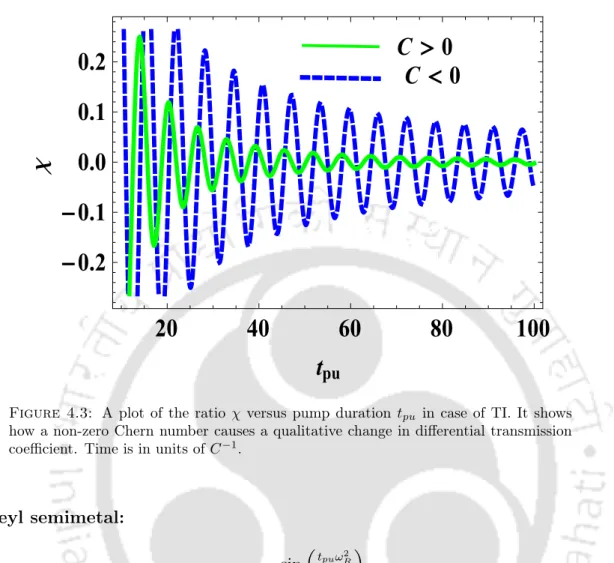Figure 4.3: A plot of the ratio χ versus pump duration t pu in case of TI. It shows how a non-zero Chern number causes a qualitative change in differential transmission coefficient

## Conclusions

The literature on Rabi oscillations and anomalous Rabi oscillations (AROs) in fermionic Dirac-Weyl systems has been well described earlier (chapter 2). In this chapter, it is shown that anomalous Rabi oscillations exist even if the EM field is treated quantum mechanically.

Problem Formulation

## Quantum Rabi Oscillations

For a field initially in the vacuum, the atomic population oscillates between excited and ground states, called vacuum field Rabi oscillations or self-induced Rabi oscillations. We study this model extensively because it captures the essence of the actual dipole coupling of an atom to an electromagnetic field.

## Jaynes Cummings Model (JCM)

The interaction between an isolated two-level atom and a single-mode quantized electromagnetic field in a lossless cavity is captured in this model. 5.4) Where Hˆ0 is the Hamiltonian of the two-level system, dˆis the dipole moment operator and E(t) is the electric field.

## Jaynes Cummings Type Model Hamiltonian for Weyl Semimetal

Due to the equality ai|d|eiˆ =hg|d|giˆ = 0 (the dipole moment is zero when the electron or hole is in the same state), and defining d = he|d|giˆ and we get d∗ = hg| d |ei, we find that dˆhas only off-diagonal elements. This model can be compared to the well-known Jaynes-Cummings model through the following identification, σ+ = σx +iσy = c†BcA; σ− = σx−iσy = c†AcB and σz = (c†AcA−c†BcB) along with a unitary transformation to photons which means that we replace bmeiωt (derived in Appendix (5.10.2)) .

## Quantum Rabi Oscillations

### Conventional Oscillations: RWA

The matrix equation obtained after diagonalization, by substitution. 5.33) Using RWA approximation we get the following equation.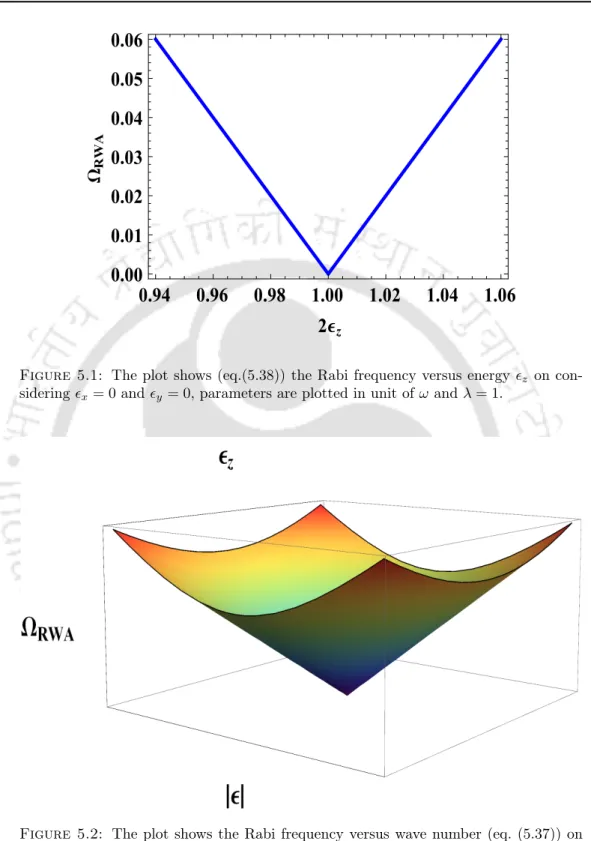Figure 5.1: The plot shows (eq.(5.38)) the Rabi frequency versus energy z on con- con-sidering x = 0 and y = 0, parameters are plotted in unit of ω and λ = 1.

### Anomalous Oscillations: ARWA

When n = 0, the smallest irregular Rabi frequency will be found, and zero photon field oscillations lead to irregular Rabi oscillations in vacuum.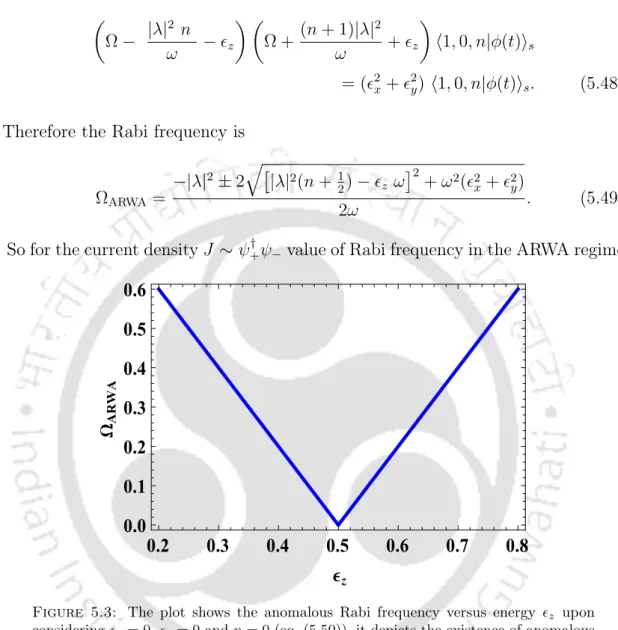Figure 5.3: The plot shows the anomalous Rabi frequency versus energy z upon considering x = 0, y = 0 and n = 0 (eq

## Numerical Solution

Third plot depicts rapid fluctuations in the above plot that make the latter appear to have a thick.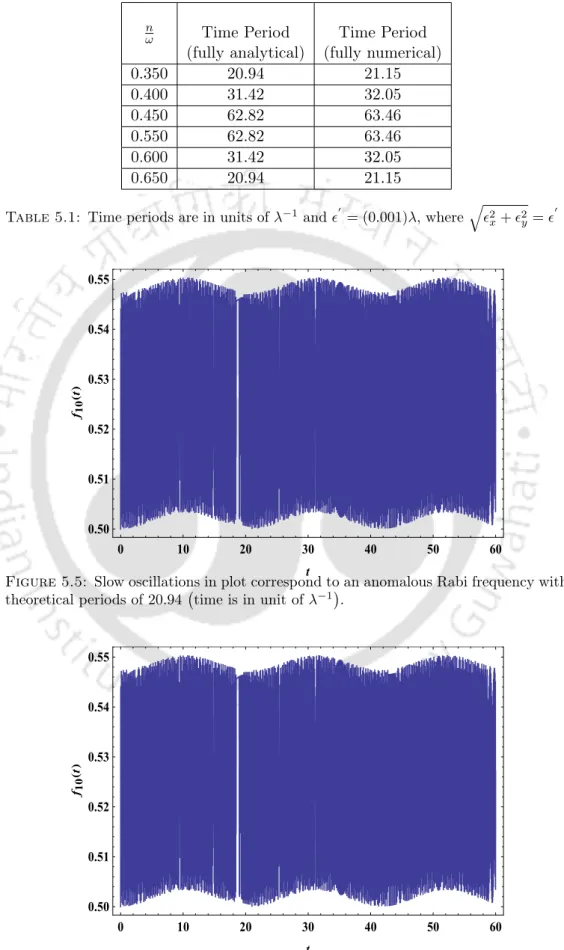Table 5.1: Time periods are in units of λ −1 and 0 = (0.001)λ, where q

Result and Discussion

## Conclusions

We have found anomalous Rabi oscillations by treating the radiation field quantum mechanically and classically. Therefore, it is not hyperbole to say that the anomalous Rabi oscillation is a phenomenon that is unequivocally suitable for studying relativistic fermionic systems.

## Appendix

### Derivation of Jaynes Cummings Type Model Hamiltonian

Dirac particles, in Weyl semimetals, in certain regions of reciprocal space, we see a shape that resembles massless Dirac particles. Thus, both the original quasi-particles and the collective mode quanta that exist in them in response to time-varying fields are relativistic in nature.

Unitary Transformation

### Bloch Equation

The phenomenon of Rabi oscillations occurs when the frequency of the incident optical field is almost equal to the transition frequency of the two-level systems, the so-called resonance condition. In this chapter we have described one of the important optical properties of relativistic fermionic systems: Rabi oscillations.

Figure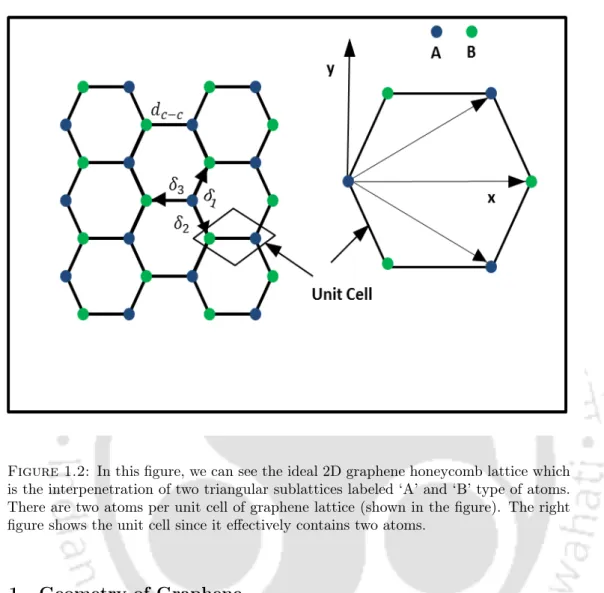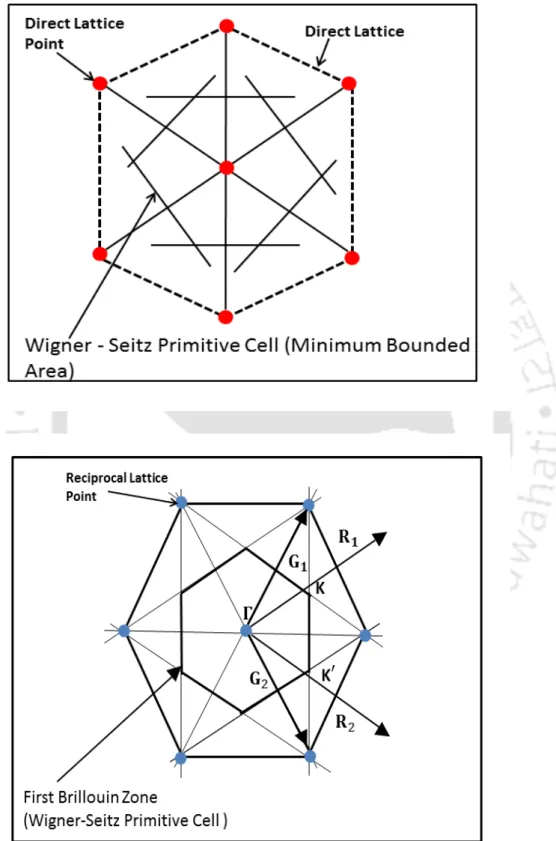+7

References

Related documents

[r]

Invasive Fungal infection in Acute Myeloid Leukemia Associated with Myeloid sarcoma of Sinonasal Cavity a rare case – Indian Journal of Otolaryngology & Head & Neck Surgery National

and Technology for Womens, Hyderabad, Telangana, India Abstract- This paper discusses the effect of a Superconducting Magnetic Energy Storage SMES on low frequency oscillations in area

In the pages that follow I will attempt the exercise of linking together a critical assessment of the role of militancy in migration studies, with the perspective suggested by the study

Passive and active by suction techniques for the control of shock wave oscillations and separated boundary layer interaction in a transonic chan- nel flow were investigated by Galli et

Which make it safe to deduced that optimum phosphorus level 60 pp/ha not only save the nitrogen to the tune of 15 kg N/ha but increase the yield by 10 per cent in radish seed production

Plan for rabi crops like chickpea and safflower Soybean  Give life saving irrigation or harvest at physiological maturity Sowing of rabi crops like sorghum, chickpea, saffalower

In this work, the dynamic avalanche model is connected with the Base push-out effect or Kirk effect for the first time to explain anomalous or non-deterministic switching behavior of

Far from conventional resonance, only relativistic fermionic systems exhibit a new type of Rabi oscillation, which is ab- sent in conventional semiconductors and two-level systems.. Our